# Test: Networks & Control Systems- 2

## 20 Questions MCQ Test GATE ECE (Electronics) 2023 Mock Test Series | Test: Networks & Control Systems- 2

Description
Attempt Test: Networks & Control Systems- 2 | 20 questions in 60 minutes | Mock test for GATE preparation | Free important questions MCQ to study GATE ECE (Electronics) 2023 Mock Test Series for GATE Exam | Download free PDF with solutions
QUESTION: 1

### For the given circuit incidence matrix A given as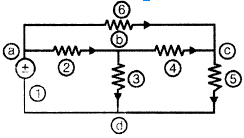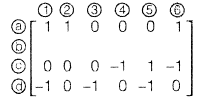This circuit has ___________ branches of tree, ________________ links and missing row in the incidence matrix is

Solution: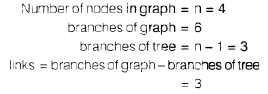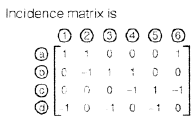QUESTION: 2

### Two inductors L1 and L2 are connected in series. The net inductance when they additively coupled is 20 H and that of oppositely coupled Is 12 H. The mutual inductance 'M' of these two is ____________ H

Solution: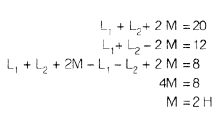QUESTION: 3

### The equivalent resistance between terminals A and B is ____________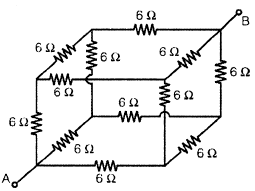Solution: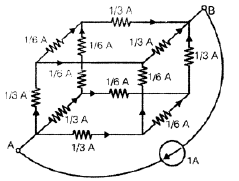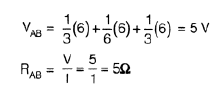QUESTION: 4

In the circuit shown in the below figure the values of E1 and E2 respectively ____________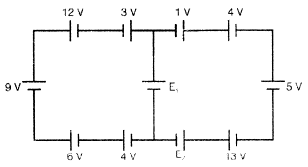Solution: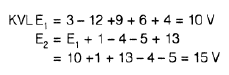QUESTION: 5

In the figure shown below V = ?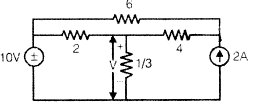Solution: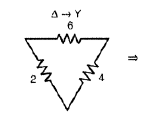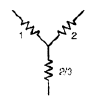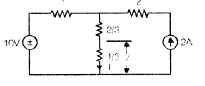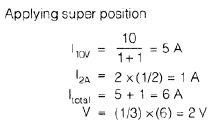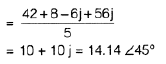QUESTION: 6

The current flowing through the branch AB is ____________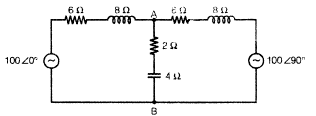Solution: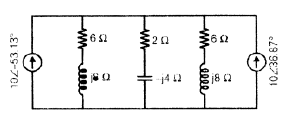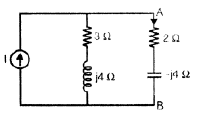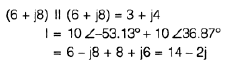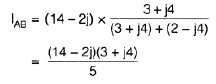QUESTION: 7

In the circuit shown in below figure VAB and ZAB respectively _______________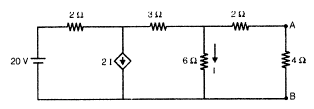Solution: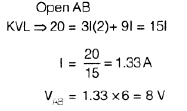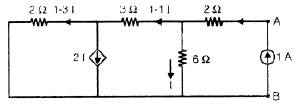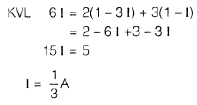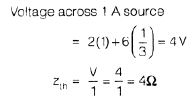QUESTION: 8

The current T in the circuit shown in below figure is ______________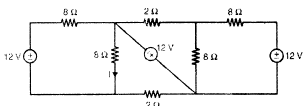Solution: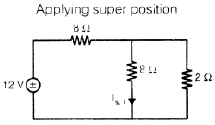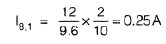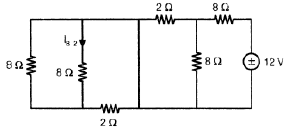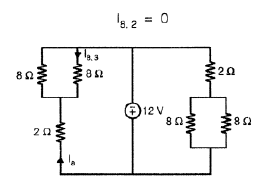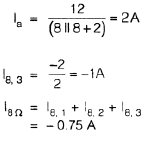QUESTION: 9

What is the value of Rto transfer maximum power to the load in the circuit shown in below figure is _________________Ω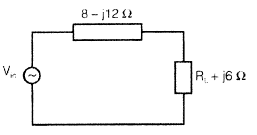Solution: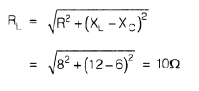QUESTION: 10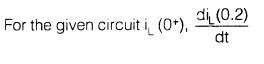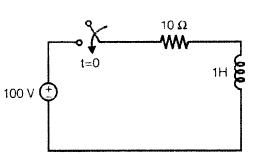Solution: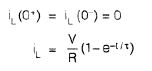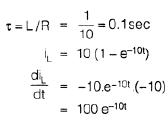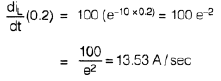QUESTION: 11

The number of forward paths and number of loops of the signal flow graph shown in below figure is _____________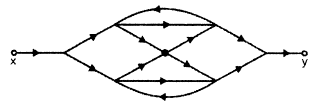Solution: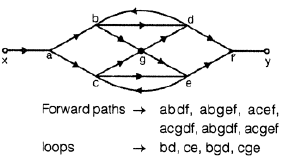QUESTION: 12

Consider the block diagram shown in the below figure.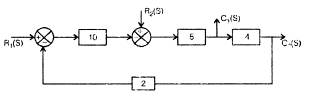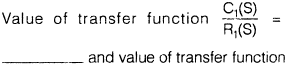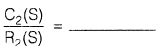Solution: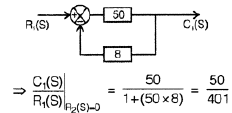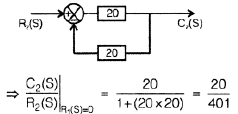QUESTION: 13

A 2nd order system has a transfer function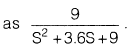In this system time required for the output to reach its 2nd peak for the unit step input is _____________ (in seconds).

Solution: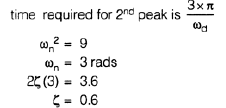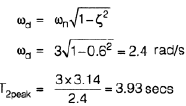QUESTION: 14

The number of poles lies in RHS of s = 1 plane in the characteristic equation s3 + 2s2 - 5s - 6 = 0 is

Solution: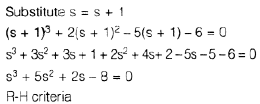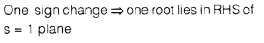QUESTION: 15

The 'root locus’ of a system is shown in the below figure. Its transfer function is _____________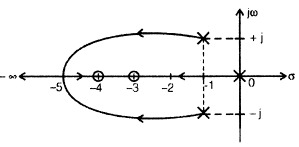Solution: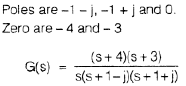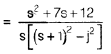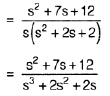QUESTION: 16

A system has open-loop transfer function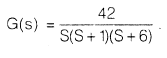Its Nyquist plot is ______________

Solution: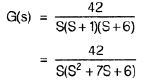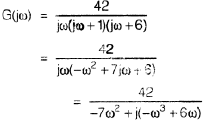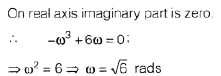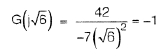QUESTION: 17

Bode plot of a transfer function is as shown in below figure.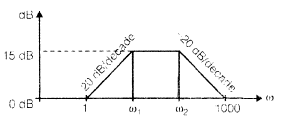The corner frequency ω1 is ___________ rad/s

Solution: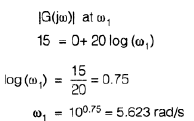QUESTION: 18

Match List-1, List-ll with List-Ill and select the correct answer using the codes given below the lists:

List-I
B.    lag compensator

List-II
Y.    transient response

List-Ill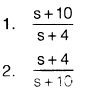Solution:
QUESTION: 19

The figure shown in below is a signal flow graph of a system. Its transfer function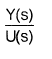is _________________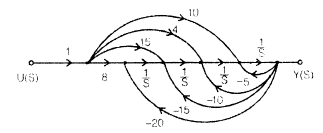Solution: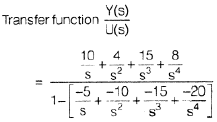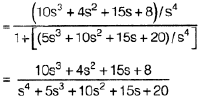QUESTION: 20

Consider the circuit shown below: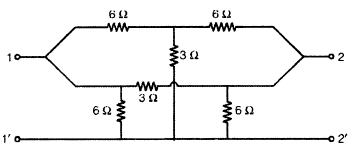The s.c. input admittance of the above network is __________________

Solution: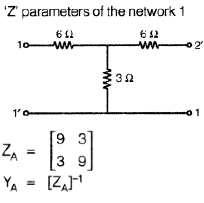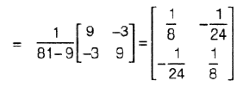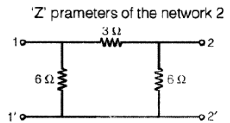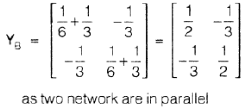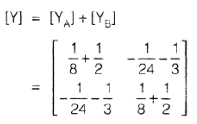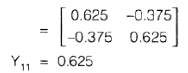Use Code STAYHOME200 and get INR 200 additional OFF Use Coupon Code